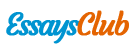# Spectrophotometric Analysis of Dyes

Autor:   •  February 5, 2019  •  1,225 Words (5 Pages)  •  445 Views

Page 1 of 5

...

[pic 3]

Figure 3. (Buret & Volumetric flask method) The Beer Law’s Plot showing the relationship between Absorbance vs concentration. The line of plot makes a curve as the concentration increases, which means the saturated points of the dye’s absorbance.

[pic 4]

Figure 2. (Buret & Volumetric flask Method) Calibration curve generated from Beer Law’s plot. Only sample 2-4 points are chosen to make calibration curve to avoid saturated sample.

Table 5. (Buret & volumetric flask method) Calculated result of slope derived from calibration curve of yellow and red dyes

Abs. Red ( λ400)

Abs. Red ( λ520)

Abs. Yellow (λ400)

Abs. Yellow (λ520)

Slope

6006.006

19019.02

16396.4

1181.181

Std. Dev.

1641.153

1385.1

5257.495

638.8656

Relative

Std. Dev (%)

27.32520204

7.28271

32.06494273

54.08700932

T-value

2.776445

2.776445

2.776445

2.776445

95% Confident Interval

2000

2000

7000

800

Calculated Value of Slope (M-1 )

6000 ± 2000

19000 ± 2000

16000 ± 7000

1200 ± 800

Table 6. (Buret & volumetric flask method) Calculated extinction coefficient ε of yellow and red at 400nm and 520nm

Wavelength (nm)

Extinction Coefficient ε (M-1 ,cm-1 )

Red

Yellow

400

6000 ± 2000

16000 ± 7000

520

19000 ± 2000

1200 ± 800

-Unknown Sports Drink (#1)

Table 7. Absorbance of unknown #1 at wavelength of 400nm and 520nm

Abs. 400nm

Abs. 520nm

Unknown #1

0.511

0.236

Table 8. Calculated Concentration of each dye in unknown #1 by 2 method

Concentration of Red dye (M)

Concentration of Yellow dye (M)

2 Syringes

(2.4± 0.9)*10-5

(5.3 ± 0.5)*10-5

(2.1± 0.7)*10-5

(5.4 ± 0.6)*10-5

Discussion

The sample set up for this experiment was prepared by two different methods: Syringe method and Buret& volumetric flask method. For syringe method, calculated concentrations of Red and Yellow dye in Unknown #1 were respectively, (2.4± 0.9)*10-5 M and (5.3 ± 0.5)*10-5M. For hhe burette method, the calculated concentrations of red and yellow dye are respectively (2.1± 0.7)*10-5 M and (5.4 ± 0.6)*10-5. As observed from these results, calculated concentration of each dye performed two methods and not much different. As a result, it is possible to say the data is very precise. However, it is not much accurate because even though a linear relationship between the concentration and the absorbance are predicted by Beer’s Law, data collected from the absorption spectra of standard solutions did not show accurate linearity, which may affect to the accuracy of the value of extinction coefficients and the calculation for concentration of unknown. Moreover, calculated uncertainty is relatively big to the mean value; also all of relative standard deviation of slopes are bigger than 5%. This can be ultimately resulting in large error from the actual concentrations after calculation. This high level of uncertainly maybe caused from dilution process of each dye to 5.0*10-5 M. To make each dye approximately 5.0*10-5 M, I supposed to dilute 1.34mL of 0.00187M red dye to 50mL, and 1.35 mL of 0.00185M Yellow dye to 50mL.I used graduated cylinder and 50mL of volumetric flask to dilute accurately however there are still uncertainly can occur. It is not possible to measure the volume accurately to 2 decimal places with graduated cylinder. I might not deliver a volume up to exactly 2 decimal places that I supposed to add. The same thing might happen during both syringe and buret methods. Moreover, there is possibility of the dye dilution was not mixed well. Since I assumed that the sample solutions in cuvette is homogenized so there might be difference between the value I assumed to collect and actual data. If the solutions are well homogenized, this clearly indicates a systematic error from how well the dilution were performed.

There is a tendency that more concentrated solution absorbs more light in Figure 1 and 3. However, it is necessary to properly remove some data point to generate calibration curve. I removed data points at the end of the curve because

...